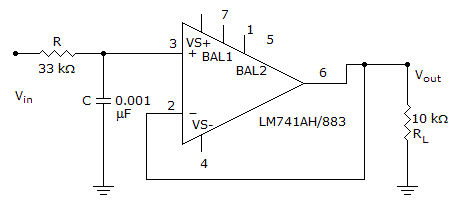# Electronics - Operational Amplifiers

### Exercise :: Operational Amplifiers - General Questions

16.

How many leads does the TO-5 metal can package of an operational amplifier have?

 A. 8, 10, or 12 B. 6, 8, or 10 C. 8 or 14 D. 8 or 16

Explanation:

No answer description available for this question. Let us discuss.

17.

If a noninverting amplifier has an RIN of 1000 ohms and an RFB of 2.5 kilohms, what is the RIN voltage when 1.42 mV is applied to the correct input?

 A. 3.5 mV B. ground C. 1.42 mV D. 0.56 mV

Explanation:

No answer description available for this question. Let us discuss.

18.

Input impedance [Zin(I)] of an inverting amplifier is approximately equal to:

 A. Ri B. Rf + Ri C. ∞ D. Rf – Ri

Explanation:

No answer description available for this question. Let us discuss.

19.

The closed-loop voltage gain of an inverting amplifier equals:

 A. the ratio of the input resistance to the feedback resistance B. the open-loop voltage gain C. the feedback resistance divided by the input resistance D. the input resistance

Explanation:

No answer description available for this question. Let us discuss.

20.

What is the cutoff frequency of this low-pass filter?A. 4.8 kHz B. 3.8 kHz C. 2.8 kHz D. 1.8 kHz